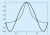# Numerical Methods for Engineers

Free!

language English Optional, paid

The development of fast, efficient and inexpensive computers has significantly increased the range of engineering problems that can be solved reliably. Numerical Methods use computers to solve problems by step-wise, repeated and iterative solution methods, which would otherwise be tedious or unsolvable by hand-calculations. This course is designed to give an overview of numerical methods of interest to scientists and engineers. However, the focus being on the techniques themselves, rather than specific applications, the contents should be relevant to varied fields such as engineering, management, economics, etc.

COURSE LAYOUT

Week-1: Introduction & Approximations

Motivation and Applications

Accuracy and precision; Truncation and round-off errors; Binary Number System; Error propagation

Week-2: Linear Systems and Equations

Matrix representation; Cramer’s rule; Gauss Elimination; Matrix Inversion; LU Decomposition;

Week-3: Linear Systems and Equations

Iterative Methods; Relaxation Methods; Eigen Values

Week-4: Algebraic Equations: Bracketing Methods

Introduction to Algebraic Equations

Bracketing methods: Bisection, Reguli-Falsi;

Week-5: Algebraic Equations: Open Methods

Secant; Fixed point iteration; Newton-Raphson; Multivariate Newton’s method

Week-6: Numerical Differentiation

Numerical differentiation; error analysis; higher order formulae

Week-7: Integration and Integral Equations

Week-8: Regression

Linear regression; Least squares; Total Least Squares;

Week-9: Interpolation and Curve Fitting

Interpolation; Newton’s Difference Formulae; Cubic Splines

Week-10: ODEs: Initial Value Problems

Introduction to ODE-IVP

Euler’s methods; Runge-Kutta methods; Predictor-corrector methods;

Week-11: ODE-IVP (Part-2)

Extension to multi-variable systems; Adaptive step size; Stiff ODEs

Week-12: ODEs: Boundary Value Problems

Shooting method; Finite differences; Over/Under Relaxation (SOR).

## User Reviews

0.0 out of 5
0
0
0
0
0

There are no reviews yet.

×